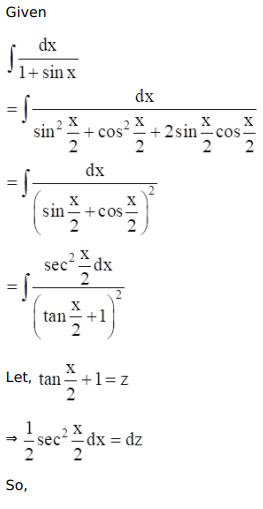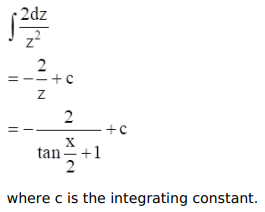# Mark against the correct answer in each of the following:

Question:

Mark $(\sqrt{)}$ against the correct answer in each of the following:

$\int \frac{\mathrm{dx}}{(1+\sin x)}=?$

A. $\tan x+\sec x+C$

B. $\tan x-\sec x+C$

C. $\frac{1}{2} \tan \frac{x}{2}+C$

D. none of these

Solution: